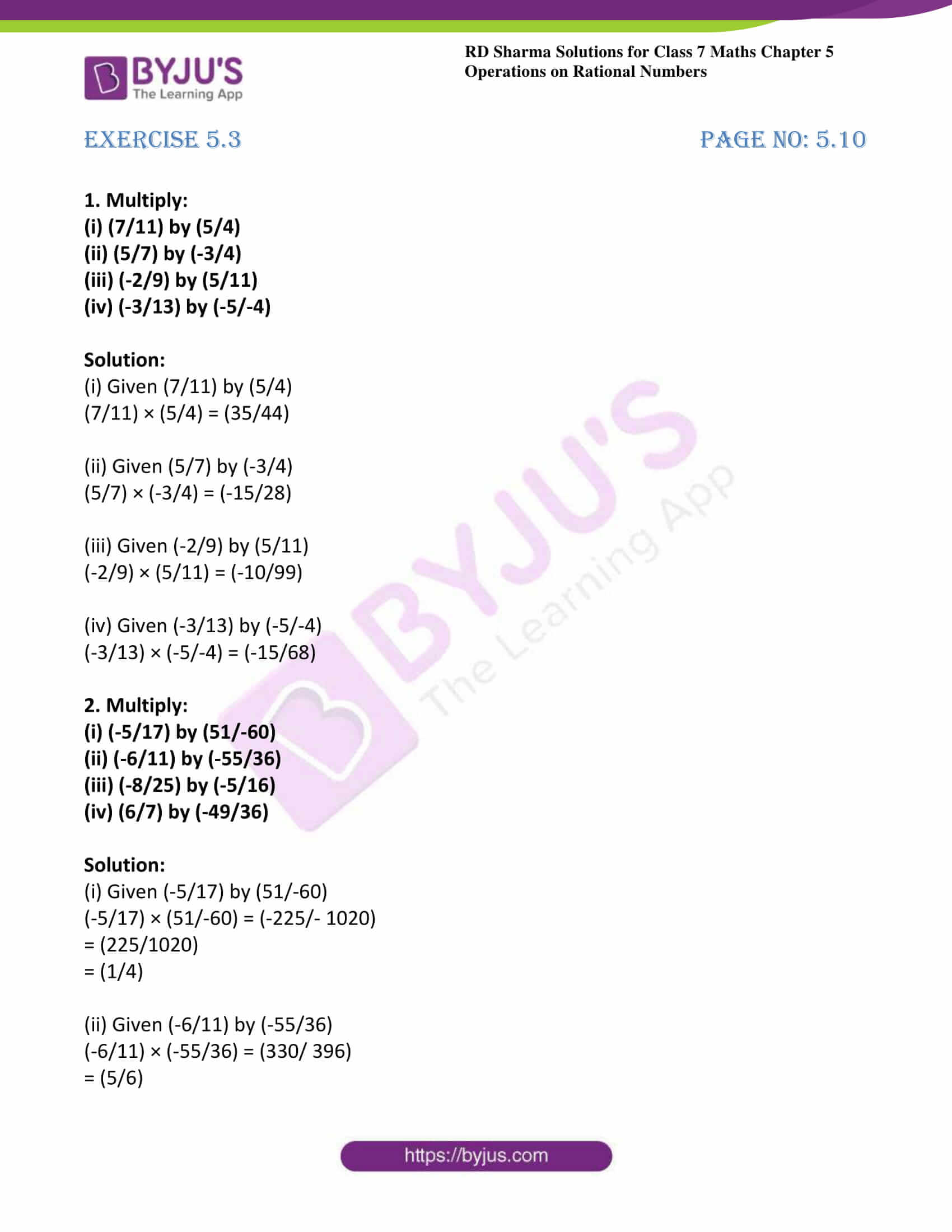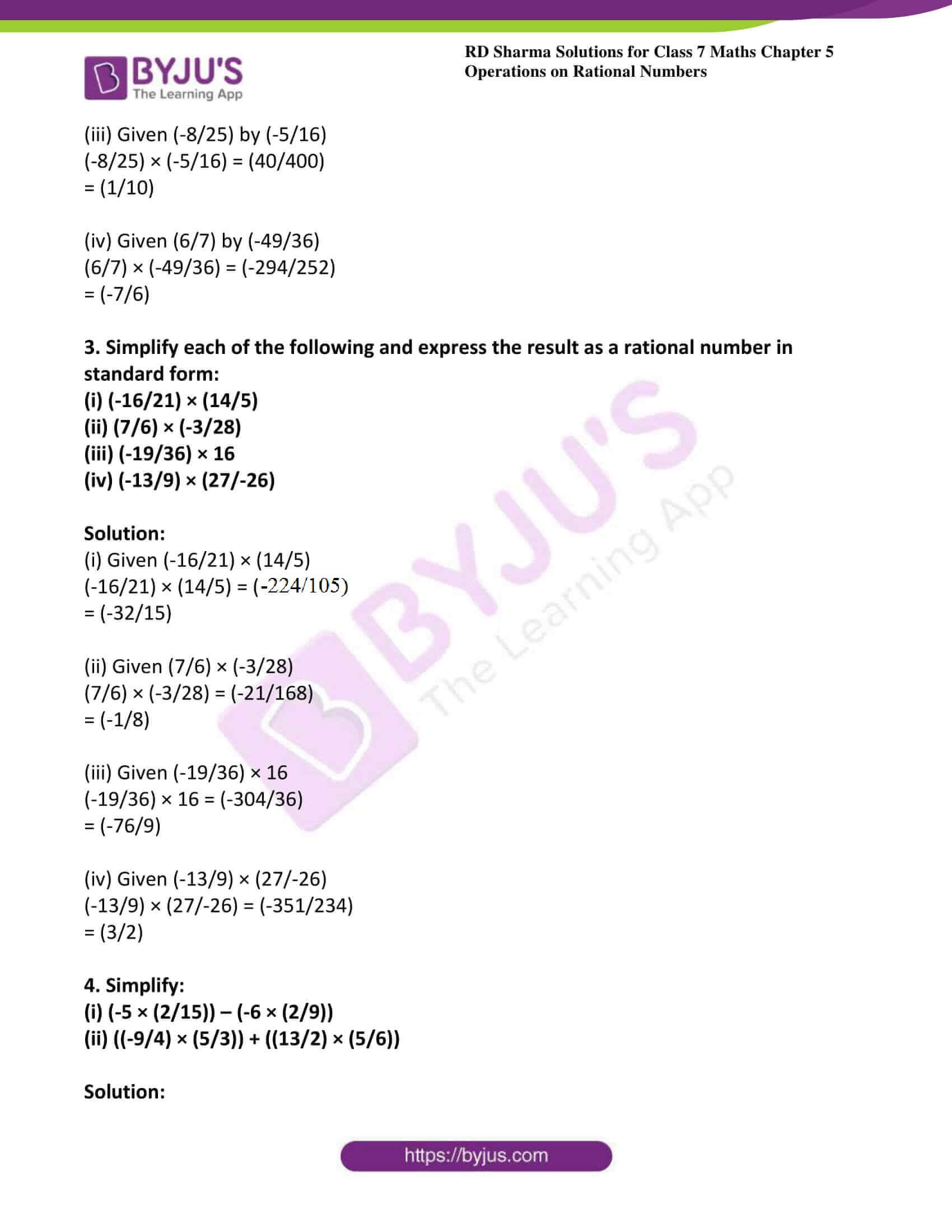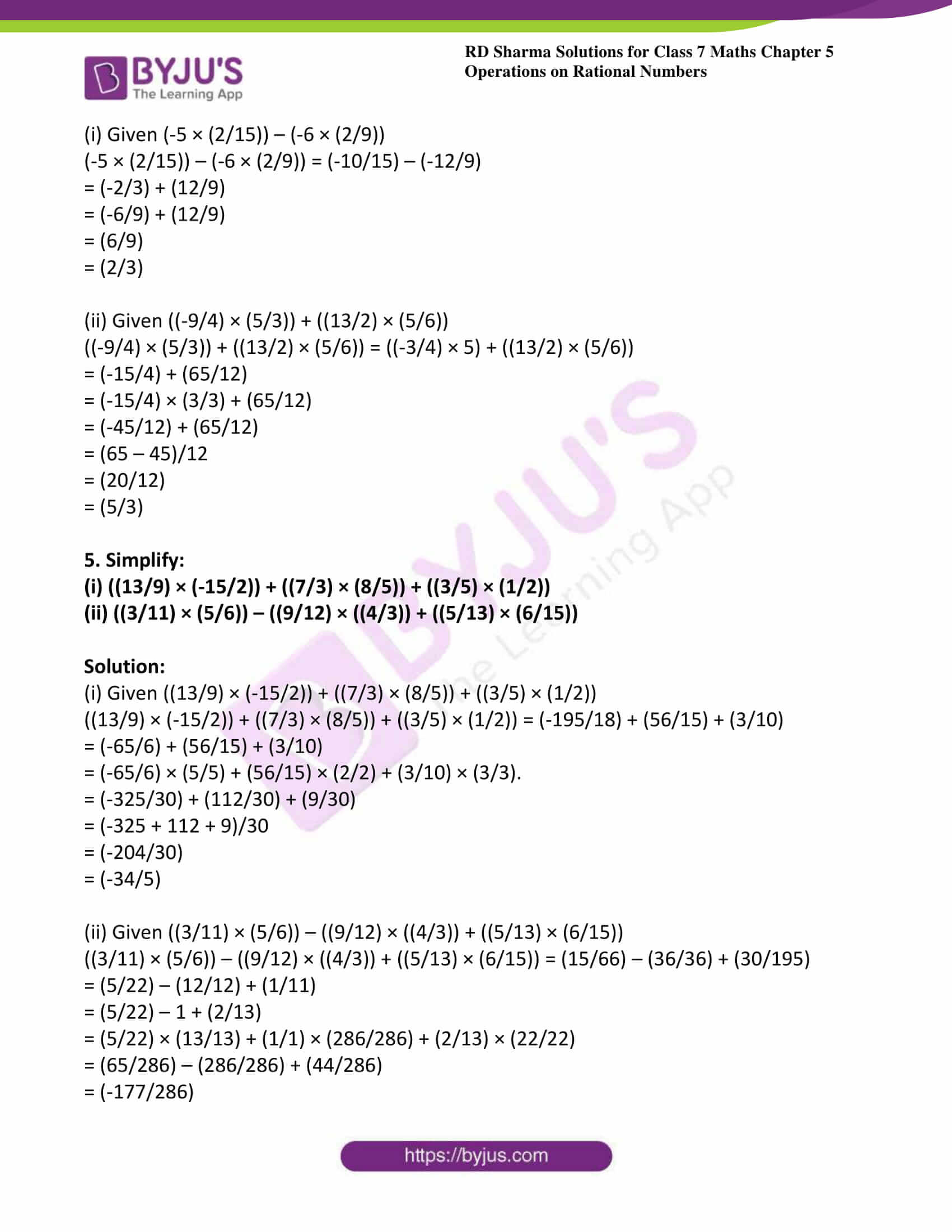# RD Sharma Solutions For Class 7 Maths Exercise 5.3 Chapter 5 Operations On Rational Numbers

Students can get the PDF of RD Sharma Solutions for Class 7 Exercise 5.3 of Chapter 5 Operations on Rational Numbers from the given links. The solutions to this exercise have formulated by BYJU’S experts in Maths to help students by clearing their doubts. In this exercise, students will learn about the multiplication of rational numbers. The rule for the product of rational numbers is as same as rule for the product of fractions that they learnt in the previous chapter. This exercise contains five questions with many sub-questions. By practising RD Sharma Solutions for Class 7 students will able to solve problems without any difficulties.

## Download the PDF of RD Sharma Solutions For Class 7 Maths Chapter 5 – Operations On Rational Numbers Exercise 5.3### Access answers to Maths RD Sharma Solutions For Class 7 Chapter 5 – Operations On Rational Numbers Exercise 5.3

1. Multiply:

(i) (7/11) by (5/4)

(ii) (5/7) by (-3/4)

(iii) (-2/9) by (5/11)

(iv) (-3/13) by (-5/-4)

Solution:

(i) Given (7/11) by (5/4)

(7/11) × (5/4) = (35/44)

(ii) Given (5/7) by (-3/4)

(5/7) × (-3/4) = (-15/28)

(iii) Given (-2/9) by (5/11)

(-2/9) × (5/11) = (-10/99)

(iv) Given (-3/13) by (-5/-4)

(-3/13) × (-5/-4) = (-15/68)

2. Multiply:

(i) (-5/17) by (51/-60)

(ii) (-6/11) by (-55/36)

(iii) (-8/25) by (-5/16)

(iv) (6/7) by (-49/36)

Solution:

(i) Given (-5/17) by (51/-60)

(-5/17) × (51/-60) = (-225/- 1020)

= (225/1020)

= (1/4)

(ii) Given (-6/11) by (-55/36)

(-6/11) × (-55/36) = (330/ 396)

= (5/6)

(iii) Given (-8/25) by (-5/16)

(-8/25) × (-5/16) = (40/400)

= (1/10)

(iv) Given (6/7) by (-49/36)

(6/7) × (-49/36) = (-294/252)

= (-7/6)

3. Simplify each of the following and express the result as a rational number in standard form:

(i) (-16/21) × (14/5)

(ii) (7/6) × (-3/28)

(iii) (-19/36) × 16

(iv) (-13/9) × (27/-26)

Solution:

(i) Given (-16/21) × (14/5)

(-16/21) × (14/5) = (-224/105)

= (-32/15)

(ii) Given (7/6) × (-3/28)

(7/6) × (-3/28) = (-21/168)

= (-1/8)

(iii) Given (-19/36) × 16

(-19/36) × 16 = (-304/36)

= (-76/9)

(iv) Given (-13/9) × (27/-26)

(-13/9) × (27/-26) = (-351/234)

= (3/2)

4. Simplify:

(i) (-5 × (2/15)) – (-6 × (2/9))

(ii) ((-9/4) × (5/3)) + ((13/2) × (5/6))

Solution:

(i) Given (-5 × (2/15)) – (-6 × (2/9))

(-5 × (2/15)) – (-6 × (2/9)) = (-10/15) – (-12/9)

= (-2/3) + (12/9)

= (-6/9) + (12/9)

= (6/9)

= (2/3)

(ii) Given ((-9/4) × (5/3)) + ((13/2) × (5/6))

((-9/4) × (5/3)) + ((13/2) × (5/6)) = ((-3/4) × 5) + ((13/2) × (5/6))

= (-15/4) + (65/12)

= (-15/4) × (3/3) + (65/12)

= (-45/12) + (65/12)

= (65 – 45)/12

= (20/12)

= (5/3)

5. Simplify:

(i) ((13/9) × (-15/2)) + ((7/3) × (8/5)) + ((3/5) × (1/2))

(ii) ((3/11) × (5/6)) – ((9/12) × ((4/3)) + ((5/13) × (6/15))

Solution:

(i) Given ((13/9) × (-15/2)) + ((7/3) × (8/5)) + ((3/5) × (1/2))

((13/9) × (-15/2)) + ((7/3) × (8/5)) + ((3/5) × (1/2)) = (-195/18) + (56/15) + (3/10)

= (-65/6) + (56/15) + (3/10)

= (-65/6) × (5/5) + (56/15) × (2/2) + (3/10) × (3/3).

= (-325/30) + (112/30) + (9/30)

= (-325 + 112 + 9)/30

= (-204/30)

= (-34/5)

(ii) Given ((3/11) × (5/6)) – ((9/12) × ((4/3)) + ((5/13) × (6/15))

((3/11) × (5/6)) – ((9/12) × ((4/3)) + ((5/13) × (6/15)) = (15/66) – (36/36) + (30/195)

= (5/22) – (12/12) + (1/11)

= (5/22) – 1 + (2/13)

= (5/22) × (13/13) + (1/1) × (286/286) + (2/13) × (22/22)

= (65/286) – (286/286) + (44/286)

= (-177/286)